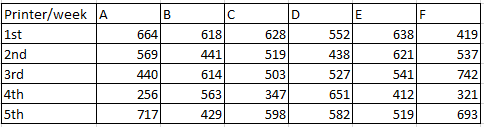# SBI PO 2017

Instructions

Study the following table carefully and answer the questions.Question 11

Question 12

Question 13

Question 14

Question 15

# What is the difference between the total number of pages printed by the Printer E in 1st, 2nd and 4th week together and total number of pages printed by Printer C in all the given weeks together.

Instructions

In each of these question two equations I & II with variables a & b are given You have to solve both the equations to find the values of a & b
a) a<b

b) $$a\leq b$$

c) relationship between a & b cannot be established

d) a>b

e) $$a\geq b$$

Question 16

Question 17

Question 18

Question 19

Question 20

OR